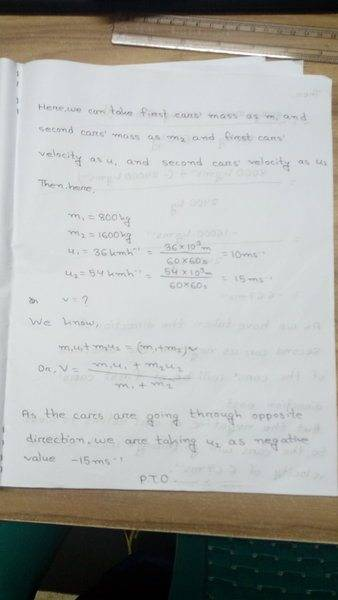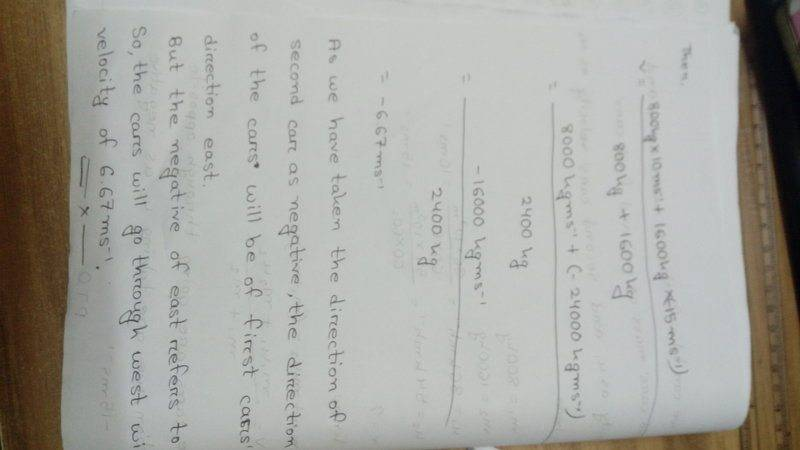# Collision of Two Cars

In summary, the two cars, with masses 800 kg and 1600 kg, respectively, were traveling towards each other at speeds of 36km/h and 54km/h. After the collision, they will move in the same direction with a velocity of 24km/h. This can be determined using the formula $m_1u_1+m_2u_2 = (m_1+m_2)v$, where $m_1$ and $m_2$ are the masses of the cars, $u_1$ and $u_2$ are their initial velocities, and $v$ is the final combined velocity.f

## Homework Statement

A car of 800 kg was going towards east with a velocity of 36km/h and an another car of 1600 kg was going towards west with a velocity of 54km/h. After collision, in which direction and with how much velocity will the cars go?

## Homework Equations

$m_1u_1+m_2u_2 = (m_1+m_2)v$

## The Attempt at a Solution

Here, we can take the first cars' mass as 1 and the second car as 2
Then,
$m_1=800kg$
$m_2=1600kg$
$u_1=36km/h$
$=\frac{36*10^3}{60*60}$
$=10m/s$
$u_2=54km/h$
$=\frac{54*10^3}{60*60}$
$=15m/s$

We know,
$m_1u_1+m_2u_2 = (m_1+m_2)v$
$Or, v = \frac{m_1u_1+m_2u_2}{m_1+m_2}$
$= \frac{800*10+1600*15}{800+1600}$
$= 13.33 m/s$ (ans.)

Last edited by a moderator:

## Homework Statement

A car of 800 kg was going towards east with a velocity of 36km/h and an another car of 1600 kg was going towards west with a velocity of 54km/h. After collision, in which direction and with how much velocity will the cars go?

## Homework Equations

$m_1u_1+m_2u_2 = (m_1+m_2)v$

## The Attempt at a Solution

Here, we can take the first cars' mass as 1 and the second car as 2
Then,
$m_1=800kg$
$m_2=1600kg$
$u_1=36km/h$
$=\frac{36*10^3}{60*60}$
$=10m/s$
$u_2=54km/h$
$=\frac{54*10^3}{60*60}$
$=15m/s$

We know,
$m_1u_1+m_2u_2 = (m_1+m_2)v$
$Or, v = \frac{m_1u_1+m_2u_2}{m_1+m_2}$
$= \frac{800*10+1600*15}{800+1600}$
$= 13.33 m/s$ (ans.)

[/B]
Can't we use LaTex here?

## Homework Statement

A car of 800 kg was going towards east with a velocity of 36km/h and an another car of 1600 kg was going towards west with a velocity of 54km/h. After collision, in which direction and with how much velocity will the cars go?

## Homework Equations

$$m_1u_1+m_2u_2 = (m_1+m_2)v$$

## The Attempt at a Solution

Here, we can take the first cars' mass as 1 and the second car as 2
Then,
$$m_1=800kg$$
$$m_2=1600kg$$
$$u_1=36km/h$$
$$=\frac{36*10^3}{60*60}$$
$$=10m/s$$
$$u_2=54km/h$$
$$=\frac{54*10^3}{60*60}$$
$$=15m/s$$

We know,
##m_1u_1+m_2u_2=(m_1+m_2)v##
##v = \frac{m_1u_1+m_2u_2}{m_1+m_2}##
$$= \frac{800*10+1600*15}{800+1600}$$ $$= 13.33 m/s$$ (ans.)[/B]
The LaTeX on this site needs double dollar signs front and back. Better, use double hash signs (#) so that it is not forced onto a new line. I've fixed it up in the copy above, but could not get one line to work.
As to your algebra, pay attention to signs. The cars were going in opposite directions.

•The LaTeX on this site needs double dollar signs front and back. Better, use double hash signs (#) so that it is not forced onto a new line. I've fixed it up in the copy above, but could not get one line to work.
As to your algebra, pay attention to signs. The cars were going in opposite directions.
Thanks a lot for your help.
Could you tell me what the answer is?

Is it right?#### Attachments

•